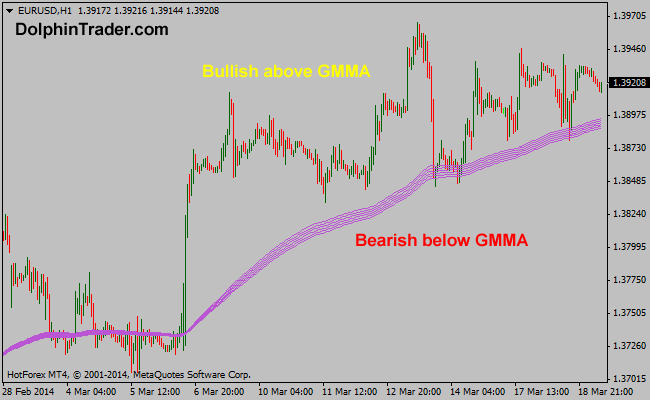# 145 EMA – 170 EMA Guppy Multi Moving Average Metatrader 4 Indicator

This is the second GMMA indicator in a series of 11 indicators entirely based on the Guppy multi moving average. The RainbowMMA_02 consists of the following moving averages: 170 period exponential moving average, 165 period exponential moving average, 160 period exponential moving average, 155 period exponential moving average, 150 period exponential moving average and 145 period exponential moving average.

Buy: Wait for the price to rise above the RainbowMMA_02 GMMA
Sell: Wait for the price to drop below the RainbowMMA_02 GMMA

Indicator Preferences

Currency pairs: any
Time frames: any
Sessions: any